/

### Enterprise Risk Management Formula Book Appendix A.2: Probability Distributions: Continuous (univariate) distributions (b) exponential, F, generalised extreme value (GEV) (and Frechét, Gumbel and Weibull)

Distribution name

Exponential distribution

Common notation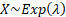Parameters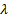= inverse scale (i.e. rate) parameter (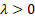)

Domain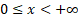Probability density function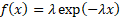Cumulative distribution function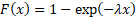Mean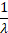Variance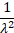Skewness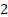(Excess) kurtosis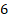Characteristic function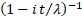Also called the negative exponential distribution. The mode of an exponential distribution is 0. The exponential distribution describes the time between events if these events follow a Poisson process. It is not the same as the exponential family of distributions. The quantile function, i.e. the inverse cumulative distribution function, is.

The non-central moments (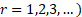are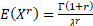. Its median is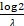.

 Distribution name F distribution Common notation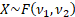Parameters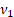= degrees of freedom (first) (positive integer)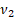= degrees of freedom (second) (positive integer) DomainProbability density function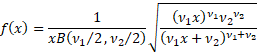Cumulative distribution function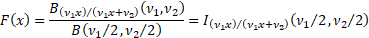Mean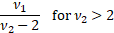Variance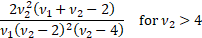Skewness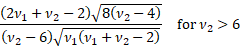(Excess) kurtosis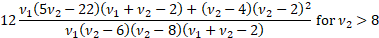Characteristic function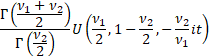Where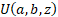is the confluent hypergeometric function of the second kind Other comments The F distribution is a special case of the Pearson type 6 distribution. It is also known as Snedecor’s F or the Fisher-Snedecor distribution. It commonly arises in statistical tests linked to analysis of variance.   If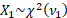and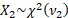are independent random variables then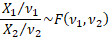The F-distribution is a particular example of the beta prime distribution.   The mode is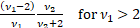. There is no simple closed form for the median.

 Distribution name Generalised extreme value (GEV) distribution (for maxima) Common notation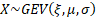Parameters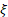= shape parameter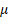= location parameter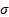= scale parameter Domain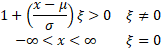Probability density function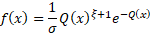where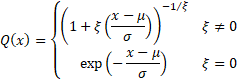Cumulative distribution function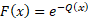Mean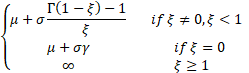where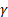is Euler’s constant, i.e.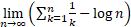Variance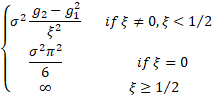Where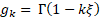Skewness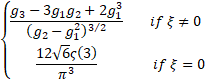where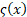is the Riemann zeta function, i.e.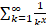. (Excess) kurtosis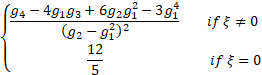Other commentsdefines the tail behaviour of the distribution. The sub-families defined by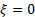(Type I),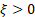(Type II) and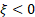(Type III) correspond to the Gumbel, Frechét and Weibull families respectively.   An important special case when analysing threshold exceedances involves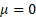(and normally) and this special case may be referred to as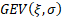.